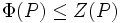# Frattini subgroup contained in center implies derived subgroup is elementary abelian

## Statement

Suppose$P$ is a group of prime power order: in other words,$P$ has order$p^n$ for some prime$p$ and integer$n$. Further, suppose that the Frattini subgroup of$P$ is contained in the center of$P$; in symbols:$\Phi(P) \le Z(P)$

Then the commutator subgroup (or derived subgroup) of$P$ is elementary Abelian.

## Facts used

1. For a group of prime power order, any subgroup containing the Frattini subgroup, has a quotient which is an elementary Abelian group
2. For a group of prime power order, the derived subgroup is contained in the Frattini subgroup. For full proof, refer: Nilpotent implies derived in Frattini
3. In a group of nilpotence class two, the map$y \mapsto [x,y]$, for fixed$x$, is an endomorphism. For full proof, refer: Class two implies commutator map is endomorphism

## Proof$P' \le \Phi(P)$, and since$\Phi(P) \le Z(P)$, and$Z(P)$ is Abelian,$P'$ is Abelian. Hence, to show that it is elementary Abelian, it suffices to show that it is generated by elements of order$p$.

We know that$P'$ is generated by commutators, i.e. elements of the form$[x,y]$ where$x,y \in P$, so it suffices to show that any commutator has order$P$.

Let's do this. Since$P' \le Z(P)$, the group$P$ has nilpotence class two. By fact (2), the map$y \mapsto [x,y]$ is, for fixed$x$, an endomorphism of$P$. Thus, we have:$[x,y^p] = [x,y]^p$$Z(P)$ contains$\Phi(P)$, so by fact (1), the quotient$P/Z(P)$ is elementary Abelian. Equivalently, for any$y \in P$, the element$y^p \in Z(P)$. Thus, the left side in the above equation is the identity element, showing that forany$x,y \in G$,$[x,y]^p = e$, completing the proof.